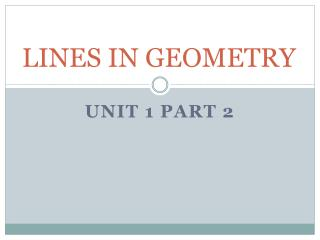DownloadDownload PresentationLINES IN GEOMETRY

LINES IN GEOMETRY

Télécharger la présentationLINES IN GEOMETRY

- - - - - - - - - - - - - - - - - - - - - - - - - - - E N D - - - - - - - - - - - - - - - - - - - - - - - - - - -
Presentation Transcript

1. LINES IN GEOMETRY Unit 1 Part 2

2. m n Perpendicular Lines Definition: Perpendicular lines are two lines that intersect to form a right angle. The symbol used for perpendicular lines is . 4 right angles are formed. In this figure line m is perpendicular to line n. With symbols we denote, m n

3. Conditional Statement Definition: A conditional statement is a statement that can be written in if-then form. “If _____________, then ______________.” Iftwo angles are adjacent and add up to 90 degrees, then the angles are complementary. Example: Continued……

4. Conditional Statement - continued Conditional Statements have two parts: The hypothesis is the part of a conditional statement that follows “if” (when written in if-then form.) The hypothesis is the given information, or the condition. The conclusionis the part of an if-then statement that follows “then” (when written in if-then form.) The conclusion is the result of the given information.

5. Three Conditional Statements • If two lines are perpendicular, then they form congruent adjacent angles. • If two lines form congruent adjacent angles, then the lines are perpendicular. The first two are converses of each other. The converse is formed by interchanging the hypothesis and the conclusion. • If the exterior sides of two adjacent acute angles are perpendicular, then the angles are complementary.

6. Parallel Lines • Parallel lines are coplanar lines that do not intersect. • Arrows are used to indicate lines are parallel. • The symbol used for parallel lines is ||. In the above figure, the arrows show that line AB is parallel to line CD. With symbols we denote, .

7. Skew Lines and Parallel Planes • Definition: Two lines are skew if they do not intersect and are not in the same plane (not coplanar). Ex: • All planes are either parallel or intersecting. • Parallel planes are two planes that do not intersect. Ex: Plane ABC and Plane EFG

8. Examples: • Name all segments that are parallel to • Name all segments that intersect • Name all segments that are skew to • Name all planes that are parallel to plane ABC. Answers: • Segments BC, FG, & EH. • Segments DH, DC, AE & AB. • Segments CG, BF, FE, & GH. • Plane FGH.

9. Slope of Parallel and Perpendicular Lines • The slope of the non vertical line through the points and is m = The slope of a vertical line is not defined. The slope of a horizontal line is zero. Two lines are parallel if and only if they have equal slopes. Two lines are perpendicular if and only if the product of their slopes is -1 (reciprocals and opposite signs).

10. Examples Any line parallel to a line with slope has slope _____. Any line perpendicular to a line with slope has slope ___. Any line parallel to a line with slope 0 has slope _____. Any line perpendicular to a line with undefined slope has slope. Any line parallel to a line with slope 2 has slope _____. 0 Zero Slope 2

11. Find the slope of the line through the given points. Examples: • (-4, 7) and (3, 7) • (3, -1) and (3, 2) • (1, -4) and (2, 5) • (-2, 5) and (1, -1)